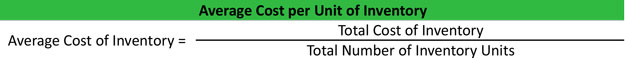# What is an Average Cost?

Definition: Average cost is a cost accounting term that is sometimes referred to as unit cost or weighted average cost. Average cost can refer to either average cost of inventory or the average cost of units produced.

These two categories are similar in nature. Retailers usually don’t produce any of their inventory; they buy it from manufacturers or wholesalers. Manufacturers, on the other hand, produce their own inventory. Retailers need to know the cost of what they paid for the inventory, while manufacturers need to know how much it costs them to produce the inventory.

## What Does Average Cost Mean?

Average is extremely easy to calculate. A retailer would calculate the average cost of inventory using the weighted average inventory method. In other words, they would divide the total dollar amount paid for the inventory by the total number of units of inventory on hand. Obviously, the total inventory must be made up the same type of units.

## Example

If a retailer averaged \$20 shirts in with \$100 shoes, the inventory average per unit would be a little skewed. Each type of inventory should be separately averaged. Here’s what the average cost formula looks like:A manufacturer’s calculation of average unit costs is just as simple as the retailers’. Take the total dollar amount spend on manufacturing a group of products and divide it by the total amount of units produced. Here is the average units equation.